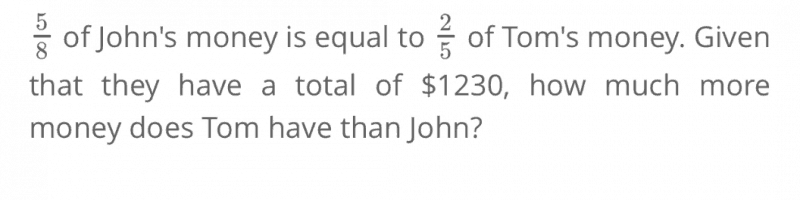# Question2 Answers

# Answer

Since 5/8 of John’s money is equal to 2/5 of Tom’s money, this means that we can try to make the numerators the same in order to compare directly.

5/8 = 10/16
2/5 = 10/25

This means that John has a total of 16 units, and Tom has a total of 25 units, for a total of 41 units and a difference of 9 units.

41 units = \$1,230
1 unit = \$1,230 ÷ 41
= 30
9 units = 9 × \$30
= \$270

0 Replies 0 Likes